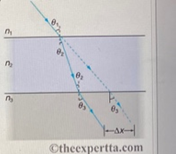# Problem: The figure on the right represents a ray of light passing from air through crown glass (which has index of refraction n = 1.52) into water, such as light beam entering a fish tank through its glass wall.Calculate the distance the ray is displaced, in millimeters, by the glass, given that the incident angle is 40.0° and the glass is 0.95 cm thick. Take 1.52 for the index of refraction for crown glass

###### FREE Expert Solution

Snell's law:

$\overline{){{\mathbf{\eta }}}_{{\mathbf{1}}}{\mathbf{s}}{\mathbf{i}}{\mathbf{n}}{{\mathbf{\theta }}}_{{\mathbf{1}}}{\mathbf{=}}{{\mathbf{\eta }}}_{{\mathbf{2}}}{\mathbf{s}}{\mathbf{i}}{\mathbf{n}}{{\mathbf{\theta }}}_{{\mathbf{2}}}}$

θ2 = sin-1((η1sinθ1)/η2) = sin-1((1sin40.0)/(1.52)) = 25.0°

90% (211 ratings)###### Problem Details

The figure on the right represents a ray of light passing from air through crown glass (which has index of refraction n = 1.52) into water, such as light beam entering a fish tank through its glass wall.Calculate the distance the ray is displaced, in millimeters, by the glass, given that the incident angle is 40.0° and the glass is 0.95 cm thick. Take 1.52 for the index of refraction for crown glass